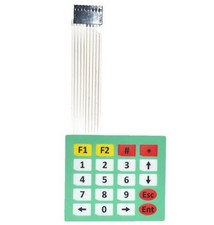Go Down

### Topic: Analog Keyboard (Read 1 time)previous topic - next topic

#### RIN67630##### May 25, 2018, 11:07 amLast Edit: May 25, 2018, 03:12 pm by RIN67630
I ever missed a decent keyboard to interact with my IOT modules.

This membrane Keyboard is dirty cheap (~1€)and provides enough possibilities for user interaction:The question was how to interface it to my modules?

The first idea was an 12C expander.
But the keyboard has got 9 lines, so I would have needed two expanders.

I followed the analog way and wired some resistors around the connector:The values are not critical, the important thing is just that the higher value resistors should ideally be ~4 times higher than the lower ones. The optimal combination is probably 1k and 4,7K but I had a mountain of 10K and 39K at hand so i used those ones.

You just need 3 wires: GND, 3,3V, and a single analog input to the arduino, no library.

The code, executed every second, is simple:

Code: [Select]
`  key = "nil";  if (A1Kyb >  310)  key = "Enter";  if (A1Kyb >  335)  key = ">";  if (A1Kyb >  350)  key = "0";  if (A1Kyb >  365)  key = "<";  if (A1Kyb >  375)  key = "Esc";  if (A1Kyb >  390)  key = "9";  if (A1Kyb >  410)  key = "8";  if (A1Kyb >  430)  key = "7";  if (A1Kyb >  443)  key = "-";  if (A1Kyb >  455)  key = "6";  if (A1Kyb >  490)  key = "5";  if (A1Kyb >  520)  key = "4";  if (A1Kyb >  537)  key = "+";  if (A1Kyb >  570)  key = "3";  if (A1Kyb >  600)  key = "2";  if (A1Kyb >  650)  key = "1";  if (A1Kyb >  672)  key = "*";  if (A1Kyb >  720)  key = "#";  if (A1Kyb >  780)  key = "F2";  if (A1Kyb >  840)  key = "F1";  Serial.print (key);  Serial.print (" A0Raw =");  Serial.println (A0Raw);`

Your mileage with the best thresholds values may vary, I just pressed every key for 5 secondes to the serial monitor, computed the thresholds and even the complete code with excel. Pated it back into The IDE and it worked out of the box.

Almost.
I had to add a 33nF capacitor between A0 and the analog GND, which stabilized the analog readings.

Another advantage is, that the circuit do not consume any current when no key is depressed, which is ideal for battery powered circuits.

Enjoy!

Go Up